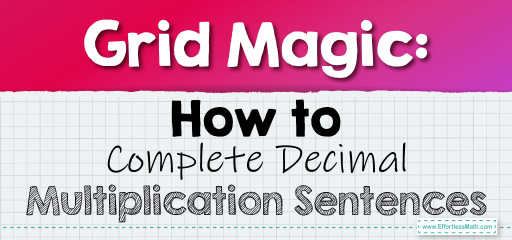# Grid Magic: How to Complete Decimal Multiplication Sentences

Grids offer a visual approach to understanding and solving decimal multiplication problems. By representing decimals as parts of a grid and using the grid to perform multiplication, we can easily complete multiplication sentences. Let's delve into this grid-based approach.Visualizing with Grids: Imagine a $$10 \times 10$$ grid where each cell represents $$0.01$$. When we talk about decimals, we can shade parts of this grid to represent them.

## Completing a Decimal Multiplication Sentence Using Grids

### Example 1:

Complete the multiplication sentence: $$0.2 \times \_ = 0.4$$.

Solution Process:

1. Shade $$20\%$$ (or $$0.2$$) of the grid.

2. Determine how many times you need to replicate this shaded portion to cover $$40\%$$ (or $$0.4$$) of the grid.

The Absolute Best Book for 5th Grade Students

Using the grid, you can determine that $$0.2$$ multiplied by $$2$$ gives $$0.4$$.

### Example 2:

Complete the multiplication sentence: $$0.5 \times \_ = 1$$.

Solution Process:

1. Shade $$50\%$$ (or $$0.5$$) of the grid.

2. Determine how many times you need to replicate this shaded portion to cover the entire grid (or $$1$$).

Using the grid, you can determine that $$0.5$$ multiplied by $$2$$ gives $$1$$.

Using grids to visualize decimal multiplication provides a tangible way to understand the concept. It bridges the gap between abstract numbers and real-world representations, making the learning process engaging and effective. Whether you’re a student, teacher, or someone looking to understand decimals better, using grids as a visual aid can be a game-changer. So, the next time you’re faced with a decimal multiplication problem, think in grids and watch the numbers come to life!

### Practice Questions:

1. Complete the multiplication sentence: $$0.3 \times \_ = 0.6$$.

2. Determine the missing factor: $$0.4 \times \_ = 0.8$$.

3. Complete the multiplication sentence: $$0.1 \times \_ = 0.5$$.

4. Determine the missing factor: $$0.6 \times \_ = 1.2$$.

5. Complete the multiplication sentence: $$0.7 \times \_ = 1.4$$.

A Perfect Book for Grade 5 Math Word Problems!

1. $$2$$

2. $$2$$

3. $$5$$

4. $$2$$

5. $$2$$

The Best Math Books for Elementary Students

### What people say about "Grid Magic: How to Complete Decimal Multiplication Sentences - Effortless Math: We Help Students Learn to LOVE Mathematics"?

No one replied yet.

X
51% OFF

Limited time only!

Save Over 51%

SAVE $15 It was$29.99 now it is \$14.99